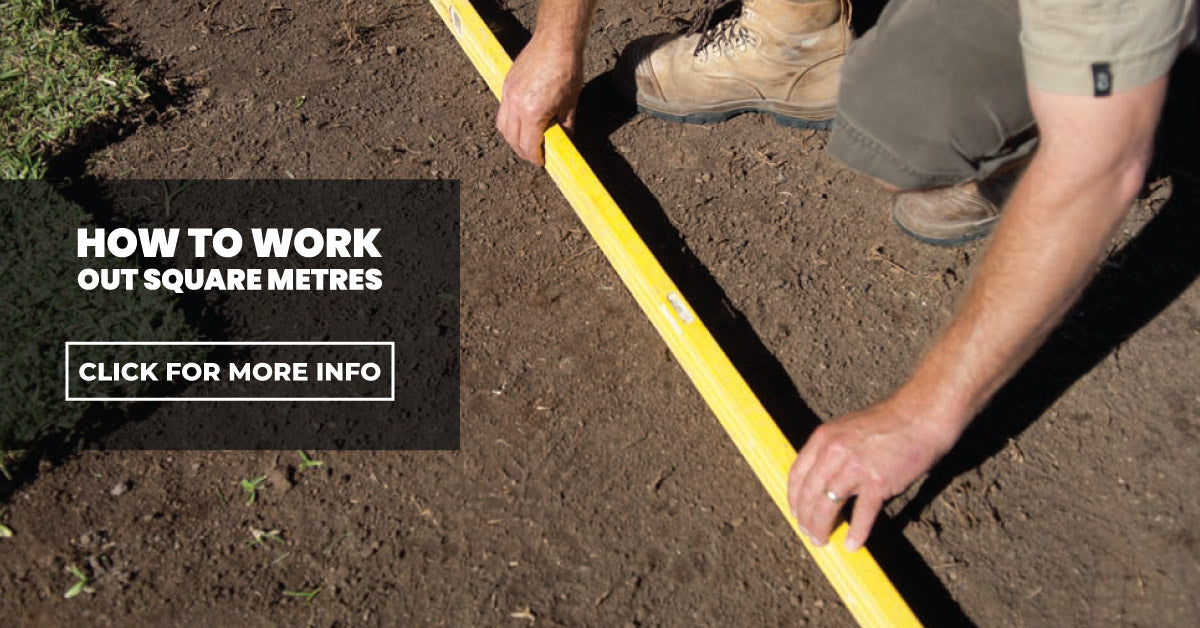Call us: (07) 3114 8281# How to work out square metres

Have you been looking to spruce up your backyard, but can’t figure out how much turf you need for the space? In this weeks article, we hope to help you work out how to calculate square meters to help you achieve your goal!

### How to measure a rectangleTo calculate how many square metres for a rectangle multiply the length x width.

Example: length 5m; width 10m

Answer: 5m x 10m = 50m²

### How to measure a circleTo calculate how many square metres for a circle multiply diameter x 0.80 then by diameter again.

Example: diameter 10m

Answer: 10m x 0.80 = 8 8 x 10m = 80m²

### How to measure an ovalTo calculate how many square metres for an oval multiply the length x 0.80 then by width.

Example: length 10m; width 7m

Answer: 10m x 0.80 x 7m = 56m²

### How to measure a triangleTo calculate how many square metres for a triangle multiply the length x width and divide by two.

Example: length 10m; width 5m

Answer: 10m x 5m = 50 ÷ 2 = 25m²

### How to measure complex shapesTo calculate how many square metres for a complex shape first divide the total area into simple shapes and calculate these areas.

Example: Rectangle: length 10m; width 7m. Triangle base 10m; height 5m

Answer: 10m x 7m = 70m² PLUS 10m x 5m = 50 ÷ 2 = 25m² TOTAL: 95m²

### How to calculate cubic metres (for soil)To calculate how many cubic metres of soil you need,
you need to multiply the length width and height.

Example: length 10m, width 10m, height 3m

Answer: 10m x 10m x 3m = 300m³

### Conclusion

It can be a hassle if you do not measure up properly before laying turf, as you may run out before you finish the job.

It is also crucial to always order extra turf, this allows you to cut in your turf properly around complex shapes and areas such as trees.

If you require any further tips on how to measure call our team on (07) 3114 8281. You can also pop in and order in person at our sales office at 1/243 Bradman Street, Acacia Ridge. The office opens Monday to Friday 7am to 5pm and Saturday 7am to 12pm. Our Acacia Ridge office also has a display area where you can kick off your shoes and try out our grasses before you buy.

45 Years

of experience in turfTurf delivered

fresh from the farmAusGAP Certified

ensures the genetic purity of our turf-grass varieties100% Secure Checkout

Zip / MasterCard / Visa